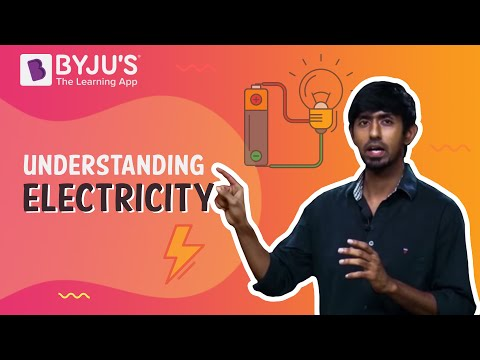# Displacement Current - Ampere-Maxwell Law

Apart from conduction current, another type of current is called displacement current. It does not appear from the real movement of electric charge, as is the case for conduction current.

## What is Displacement Current?

Displacement current is a quantity appearing in Maxwell’s equations. Displacement current definition is defined in terms of the rate of change of the electric displacement field (D). It can be explained by the phenomenon observed in a capacitor.

### Current in a capacitor.

When a capacitor starts charging, there is no conduction of charge between the plates. However, because of the change in charge accumulation with time above the plates, the electric field changes causing the displacement current as below-

$$\begin{array}{l}I_{D}=J_{D}S=S\frac{\partial D}{\partial t}\end{array}$$

Where,

• S is the area of the capacitor plate.
• ID is the displacement current.
• JD  is the displacement current density.
• D is related to electric field E as
$$\begin{array}{l}D=\varepsilon E\end{array}$$
• $$\begin{array}{l}\varepsilon \end{array}$$
is the permittivity of the medium in between the plates.

## Displacement Current Equation

Displacement current has the same unit and effect on the magnetic field as is for conduction current depicted by Maxwell’s equation-

$$\begin{array}{l}\bigtriangledown \times H = J+J_{D}\end{array}$$

Where,

• H is related to magnetic field B as
$$\begin{array}{l}B=\mu H\end{array}$$
• $$\begin{array}{l}\mu \end{array}$$
is the permeability of the medium in between the plates.
• J is the conducting current density.
• JD  is the displacement current density.

We know that

$$\begin{array}{l}\bigtriangledown .\left ( \bigtriangledown \times H \right )=0\end{array}$$
and
$$\begin{array}{l}\bigtriangledown .J=-\frac{\partial \rho }{\partial t} =-\bigtriangledown .\frac{\partial D}{\partial t}\end{array}$$
using Gauss’s law that is
$$\begin{array}{l}\bigtriangledown .D=\rho\end{array}$$

Here,

$$\begin{array}{l}\rho\end{array}$$
is the electric charge density.

Thus,

$$\begin{array}{l}J_{D}=\frac{\partial D}{\partial t}\end{array}$$
displacement current density is necessary to balance RHS with LHS of the equation.

## Displacement Current Questions

Q1: What is the SI unit of displacement current?

A: the SI unit of displacement current is Ampere (A)

Q2: How does the displacement current arise?

A: Displacement current arises due to varying electromotive force.

Q3: What is the output of a capacitor connected to displacement current?

A: Zero

Q4: What is displacement current?

A: The current due to the changing electric field is called displacement current.

Q5: Does the displacement current satisfy the property of continuity?

A: Yes, the sum of displacement and conduction current remains constant along the closed path.

Q6: Write the expression for the displacement current.

A:

$$\begin{array}{l}I_{D}=J_{D}S=S\frac{\partial D}{\partial t}\end{array}$$

Where,

• S is the area of the capacitor plate.
• ID is the displacement current.
• JD  is the displacement current density.
• D is related to electric field E as
$$\begin{array}{l}D=\varepsilon E\end{array}$$
• $$\begin{array}{l}\varepsilon \end{array}$$
is the permittivity of the medium in between the plates.

Q7: What is the magnitude of displacement current in the case of steady electric fields in a conducting wire?

A: Zero

Q8: What current exists inside a capacitor?

A: The current that exists inside the capacitor is Displacement current.

Q9: State Ampere-Maxwell law

A: The line integral of the magnetic field around a closed loop is equal to μ0 times the sum of conduction current and displacement current.

Q10: Why was the concept of displacement current introduced?

A: Displacement current was introduced to the current in terms of Ampere circuit law to make it logically consistent.

Q11: Distinguish between conduction current and displacement current?

A: Conduction current is due to the flow of electrons in a circuit. It exists even if electrons flow at a uniform rate.

Displacement current is due to the time-varying electric field. It does not exist under steady conduction.

Q12: What is the magnitude of displacement current in the case of steady electric fields in a conducting wire? Explain.

A: The magnitude of displacement current in the case of steady electric fields in a conducting wire is zero since the electric field E does not change with time.

Q13: How is the concept of displacement current associated with the behaviour of electric and magnetic fields?

A: According to Faraday’s law of electromagnetic induction, a time-varying magnetic field induces an emf, According to Maxwell, an electric field sets up a current and hence a magnetic field. Such a current is called displacement current. It follows that a time-varying electric field produces a magnetic field and vice-versa. Hence, the behaviour of the electric and magnetic field is symmetric.

Hope you have understood about Displacement current, Ampere-Maxwells law, Displacement current formula, displacement current equation, its significance, terms, and Units along with practice questions.

Physics Related Topics:

Stay tuned with BYJU’S for more such interesting articles. Also, register to “BYJU’S-The Learning App” for loads of interactive, engaging physics-related videos and an unlimited academic assistance.

## Understand Electricity in just 5 minutes!Test your knowledge on Displacement current